Anúncio

# “To estimate the charge induced on each one of the two identical Styrofoam (or pith) balls suspended in the vertical plane by making use of Coulomb’s Law”

A High School Student
1 de May de 2022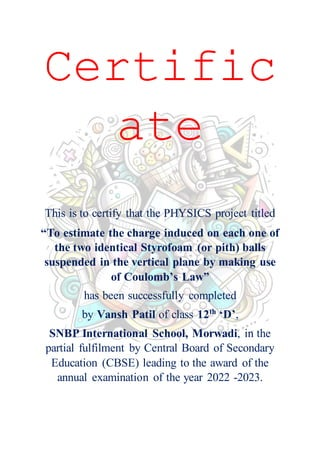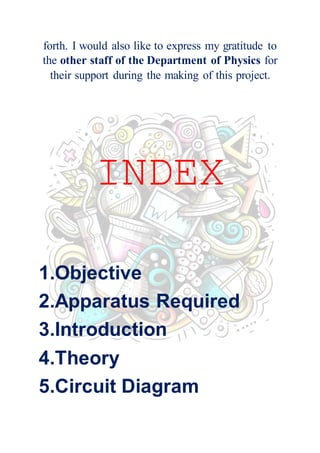Anúncio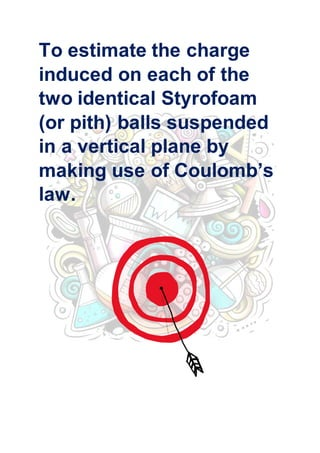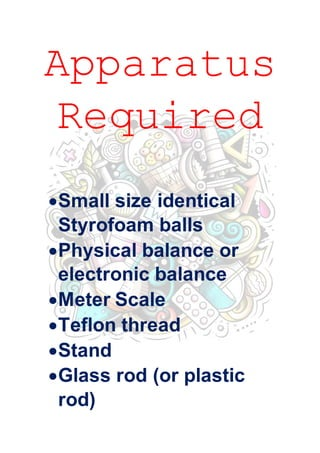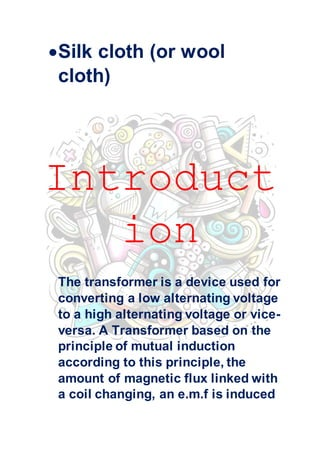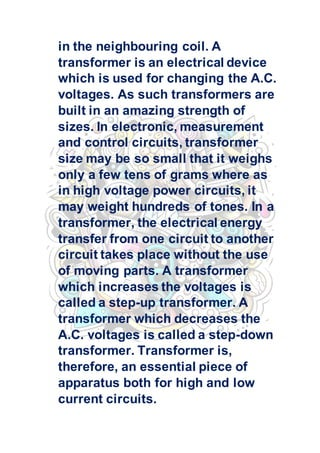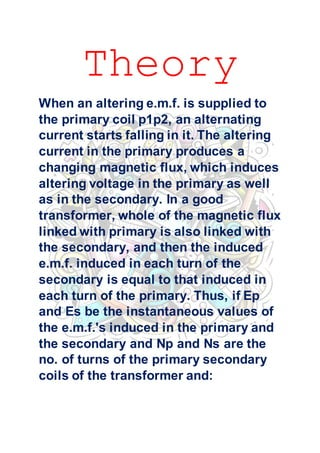Anúncio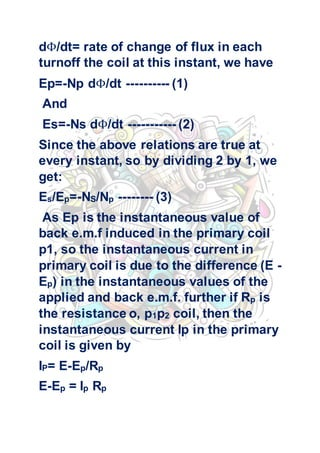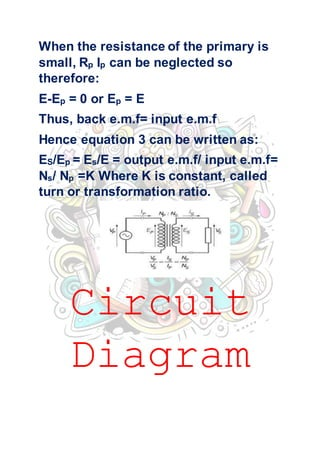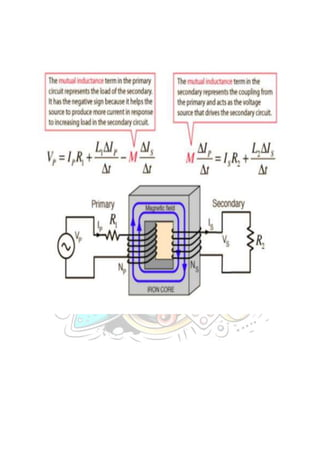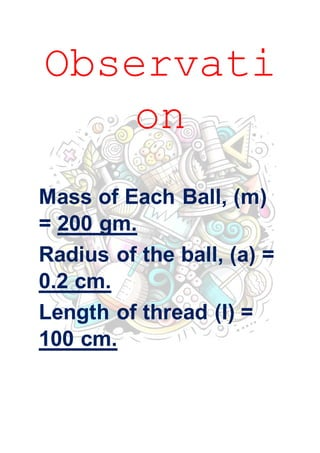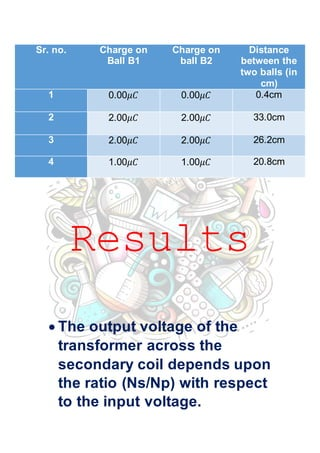Anúncio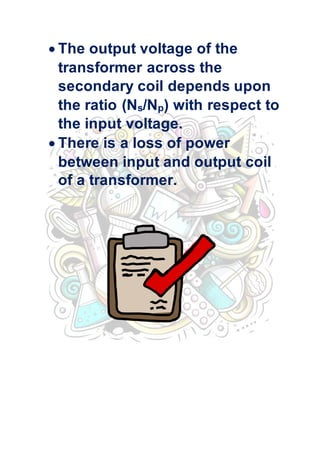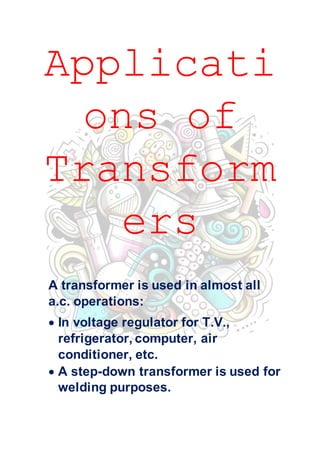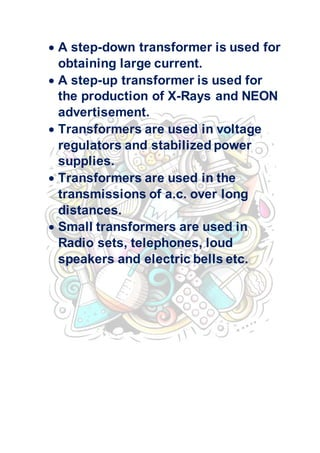AnúncioPróximos SlideShares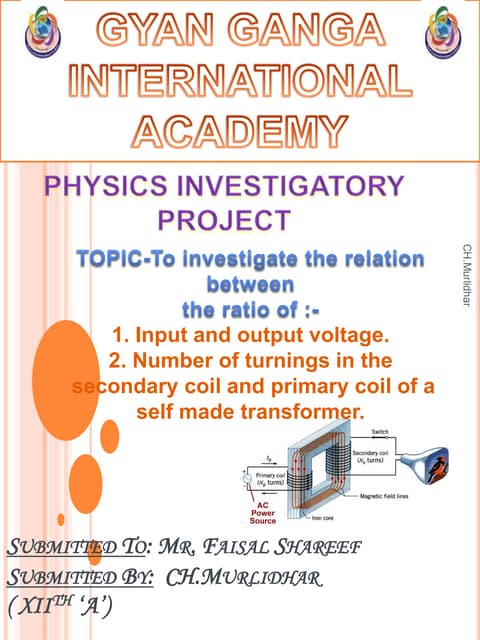TOPIC-To investigate the relation between the ratio of :- 1. Input and outpu...
Carregando em ... 3
1 de 21
Anúncio

### “To estimate the charge induced on each one of the two identical Styrofoam (or pith) balls suspended in the vertical plane by making use of Coulomb’s Law”

1. Certific ate This is to certify that the PHYSICS project titled “To estimate the charge induced on each one of the two identical Styrofoam (or pith) balls suspended in the vertical plane by making use of Coulomb’s Law” has been successfully completed by Vansh Patil of class 12th ‘D’, SNBP International School, Morwadi, in the partial fulfilment by Central Board of Secondary Education (CBSE) leading to the award of the annual examination of the year 2022 -2023.
2. ACKNOWLEDG EMENT “There are times when silence speak so much louder than words of praise to only as good as belittle a person, whose words do not express, but only put a veneer over true feelings, which are of gratitude at this point of time.” I would like to express my sincere gratitude to my physics teacher Miss Luna and Miss Ruchita, for her vital support, guidance and encouragement, without which this project would not have come
3. forth. I would also like to express my gratitude to the other staff of the Department of Physics for their support during the making of this project. INDEX 1.Objective 2.Apparatus Required 3.Introduction 4.Theory 5.Circuit Diagram
4. 6.Observations/Observati on Table 7.Results 8.Applications 9.Bibliography OBJECTIVE
5. To estimate the charge induced on each of the two identical Styrofoam (or pith) balls suspended in a vertical plane by making use of Coulomb’s law.
6. Apparatus Required Small size identical Styrofoam balls Physical balance or electronic balance Meter Scale Teflon thread Stand Glass rod (or plastic rod)
7. Silk cloth (or wool cloth) Introduct ion The transformer is a device used for converting a low alternating voltage to a high alternating voltage or vice- versa. A Transformer based on the principle of mutual induction according to this principle, the amount of magnetic flux linked with a coil changing, an e.m.f is induced
8. in the neighbouring coil. A transformer is an electrical device which is used for changing the A.C. voltages. As such transformers are built in an amazing strength of sizes. In electronic, measurement and control circuits, transformer size may be so small that it weighs only a few tens of grams where as in high voltage power circuits, it may weight hundreds of tones. In a transformer, the electrical energy transfer from one circuit to another circuit takes place without the use of moving parts. A transformer which increases the voltages is called a step-up transformer. A transformer which decreases the A.C. voltages is called a step-down transformer. Transformer is, therefore, an essential piece of apparatus both for high and low current circuits.
9. Theory When an altering e.m.f. is supplied to the primary coil p1p2, an alternating current starts falling in it. The altering current in the primary produces a changing magnetic flux, which induces altering voltage in the primary as well as in the secondary. In a good transformer, whole of the magnetic flux linked with primary is also linked with the secondary, and then the induced e.m.f. induced in each turn of the secondary is equal to that induced in each turn of the primary. Thus, if Ep and Es be the instantaneous values of the e.m.f.'s induced in the primary and the secondary and Np and Ns are the no. of turns of the primary secondary coils of the transformer and:
10. dΦ/dt= rate of change of flux in each turnoff the coil at this instant, we have Ep=-Np dΦ/dt ---------- (1) And Es=-Ns dΦ/dt ----------- (2) Since the above relations are true at every instant, so by dividing 2 by 1, we get: Es/Ep=-NS/Np -------- (3) As Ep is the instantaneous value of back e.m.f induced in the primary coil p1, so the instantaneous current in primary coil is due to the difference (E - Ep) in the instantaneous values of the applied and back e.m.f. further if Rp is the resistance o, p1p2 coil, then the instantaneous current Ip in the primary coil is given by IP= E-Ep/Rp E-Ep = Ip Rp
11. When the resistance of the primary is small, Rp Ip can be neglected so therefore: E-Ep = 0 or Ep = E Thus, back e.m.f= input e.m.f Hence equation 3 can be written as: ES/Ep = Es/E = output e.m.f/ input e.m.f= Ns/ Np =K Where K is constant, called turn or transformation ratio. Circuit Diagram
12. Observati on Mass of Each Ball, (m) = 200 gm. Radius of the ball, (a) = 0.2 cm. Length of thread (I) = 100 cm.
13. Results  The output voltage of the transformer across the secondary coil depends upon the ratio (Ns/Np) with respect to the input voltage. Sr. no. Charge on Ball B1 Charge on ball B2 Distance between the two balls (in cm) 1 0.00𝜇𝐶 0.00𝜇𝐶 0.4cm 2 2.00𝜇𝐶 2.00𝜇𝐶 33.0cm 3 2.00𝜇𝐶 2.00𝜇𝐶 26.2cm 4 1.00𝜇𝐶 1.00𝜇𝐶 20.8cm
14.  The output voltage of the transformer across the secondary coil depends upon the ratio (Ns/Np) with respect to the input voltage.  There is a loss of power between input and output coil of a transformer.
15. Applicati ons of Transform ers A transformer is used in almost all a.c. operations:  In voltage regulator for T.V., refrigerator, computer, air conditioner, etc.  A step-down transformer is used for welding purposes.
16.  A step-down transformer is used for obtaining large current.  A step-up transformer is used for the production of X-Rays and NEON advertisement.  Transformers are used in voltage regulators and stabilized power supplies.  Transformers are used in the transmissions of a.c. over long distances.  Small transformers are used in Radio sets, telephones, loud speakers and electric bells etc.